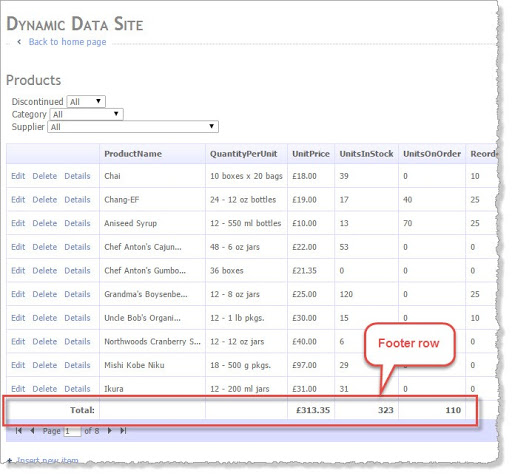## Thursday, 10 September 2015

### Automatic Totals on your List page in Dynamic Data

So the idea here is add an attribute to your table (TotalsAttribute in this case) and then have a total appear on your list page.

Note: Note this will only work on Numeric columns int, Decimal, float etc.

Figure 1 shows what we are going to achieve, the columns here don’t make sense to total but it’s only an example  for real i would add a computed column that multiplied the unit cost with the qty in stock and total that but here it’s just to show how it works with decimal and int values.Figure 1 – Footer row with totals.

### The Attribute

We will need an attribute because we want this to happen automatically and not have to create a custom page, for this I have decided to add a class level attribute called TotalsAttribute you can see the code in Listing 1.

```[AttributeUsage(AttributeTargets.Class)]public class TotalsAttribute : Attribute{    public String[] Columns { get; set; }    public TotalsAttribute()     {         Columns = new String;    }    public TotalsAttribute(params String[] columns)    {        Columns = columns;    }}
```

Listing 1 – TotalsAttribute

All we are doing here is keeping an array of column names that we want totals on, most of the time it will be single column, but it’s nice to have the option of multiple.

```[Totals("UnitPrice", "UnitsInStock", "UnitsOnOrder")][MetadataType(typeof(Product.Metadata))]public partial class Product{    internal sealed class Metadata    {        public Int32 ProductID { get; set; }        public String ProductName { get; set; }        [DataType(DataType.Currency)]        public Nullable<int> UnitPrice { get; set; }        public Nullable<int> UnitsInStock { get; set; }        //... other column removed for simplicity    }}
```

Listing 2 – example of attribute in use.

In the example [Totals("UnitPrice", "UnitsInStock", "UnitsOnOrder")] in Listing 2 we are telling the system that we want totals on three columns “UnitPrice”, “UnitsInStock”, “UnitsOnOrder”.

### The Custom Code in the List page

There are two things we need in the page first we will need to check if there are totals on this table and if so wire it all up. In Listing 3 we have all the code we need to test if there are totals for the current Table and if so wire up the Row DataBound even handler which you can see in Listing 4.

In Listing 3 we single get the attribute from the table and test to see if what we got is not null, if not we can the wire up the event handler. But also we need to turn on the footer.

!Important: I found this bit missing from most of the articles I found whilst searching for examples of how to do this; everything works without this, it just doesn't display. The bit you must have is GridView1.ShowFooter = true;
Note: I am using some custom extension methods to get the attribute, these are in the root of the application and the file is called “AttributeExtensionMethods.cs”
```public partial class List : System.Web.UI.Page
{
protected TotalsAttribute totalsAttribute;
protected MetaTable table;

protected void Page_Init(object sender, EventArgs e)
{
table = DynamicDataRouteHandler.GetRequestMetaTable(Context);
GridView1.SetMetaTable(table, table.GetColumnValuesFromRoute(Context));
GridDataSource.EntityTypeFilter = table.EntityType.Name;

// get the attribute
totalsAttribute = table.GetAttribute<TotalsAttribute>();

// if the attribute is not null then we have some totals
if (totalsAttribute != null && totalsAttribute.Columns.Count() > 0)
{
// show the footer
GridView1.ShowFooter = true;
// wire up the row data bound event
GridView1.RowDataBound += OnRowDataBound;
}
}
// rest of code behind removed for simplicity
```

Listing 3 – testing if we have any totals for this table.

Now all we need the workhorse code the stuff that is going to total up and then display the totals in the footer. See Listing 4 I have tried to put a lot of comments in there to help but here’s a brief explanation of what it does:

The code in the event handler is split into two sections one for the DataRow and one for the Footer you can see there are encased in two if statements. Also note we have a global variable “totals” this is used to keep a total of each column we are totalling and is a dictionary of Decimal values.

The DataRow

Here we iterate through the totals columns from the attribute and sum up each one, you will notice that I am testing if the column is a valid column by checking the metadata to see if it is an int or an floating point this stops us having a nasty error.

```// NOTE: if you are using a column generator (IAutoFieldGenerator)
// the this may not work if it re-orders the displayed columns
protected Dictionary<String, Decimal> totals = new Dictionary<String, Decimal>();
protected void OnRowDataBound(object sender, GridViewRowEventArgs e)
{
// this will only be wired up and called if there are totals so we don't need to test.
// Get a List<String> of column names if the order that they appear in the GridView1

// if this is a data row get the totals
if (e.Row.RowType == DataControlRowType.DataRow)
{
foreach (var column in totalsAttribute.Columns)
{
// get the MetaColumn
var metaColumn = displayedColumns.First(c => c.Name == column);

// check this column is a valid column to total i.e. int Decimal, float etc.
if (metaColumn.IsFloatingPoint || metaColumn.IsInteger)
{
// initialize variable if not present
if (!totals.ContainsKey(column))

totals[column] += Convert.ToDecimal(DataBinder.Eval(e.Row.DataItem, column));
}
}
}

// if we are on the footer row render the totals.
if (e.Row.RowType == DataControlRowType.Footer)
{

// set total description name
e.Row.Cells.Text = "Total:";
e.Row.Cells.CssClass = "right";

foreach (var column in totalsAttribute.Columns)
{
// get index of column plus offset of 1 for the command button column
var index = displayedColumns.FindIndex(c => c.Name == column) + 1;

var metaColumn = displayedColumns.First(c => c.Name == column);
if (metaColumn.IsFloatingPoint || metaColumn.IsInteger)
{
// for the Footer, display the running totals
e.Row.Cells[index].Text = metaColumn.FormatValue(totals[column]);